## unity双滑动列表实现

|     |   0 浏览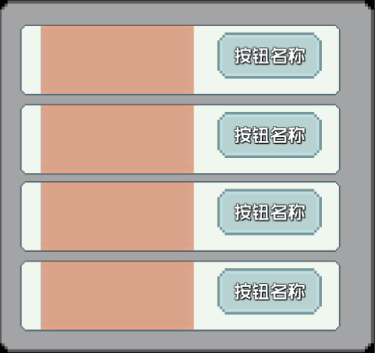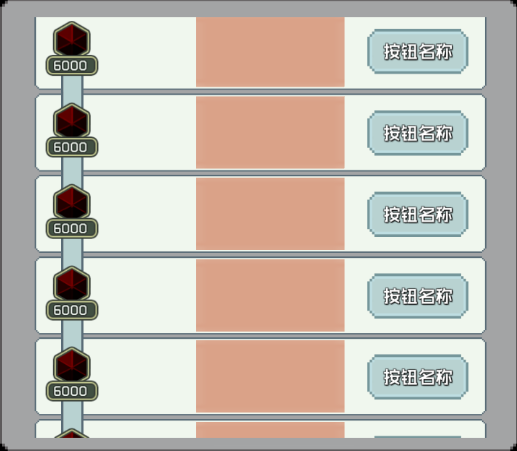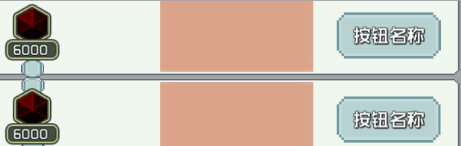#### 尝试一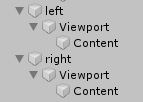``````function base.updaterender(go, index)
local posRigth = ui.list.gameObject.transform.localPosition
local posLeft = ui.proglist.gameObject.transform.localPosition
ui.proglist.gameObject.transform.localPosition = Vector2.New(posLeft.x,posRigth.y)
end
``````

#### 尝试二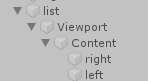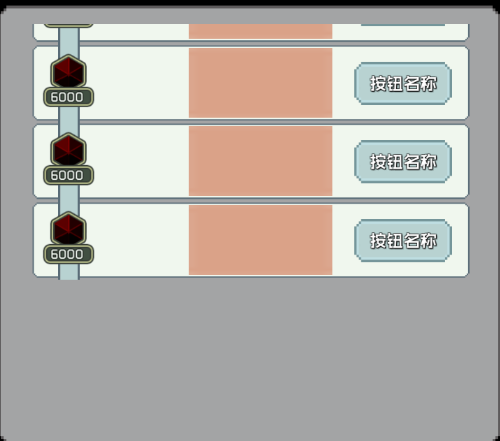``````local function SliderIdx()
local idx = FindCanGetIdx()--需要滑动到的item下标
local pos = ui.content.transform.localPosition
local posY
if idx then
posY = (idx-1)*111.35
ui.content.gameObject.transform.localPosition = Vector2.New(0, 0)
ui.content.gameObject.transform:DOLocalMove(Vector2.New(0, posY), 0.8, false):SetEase(Ease.Linear):Play()
else
ui.content.transform.localPosition = Vector2.New(ui.content.transform.localPosition.x,0)
end
end
``````

#### 尝试三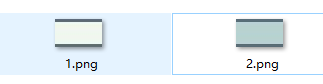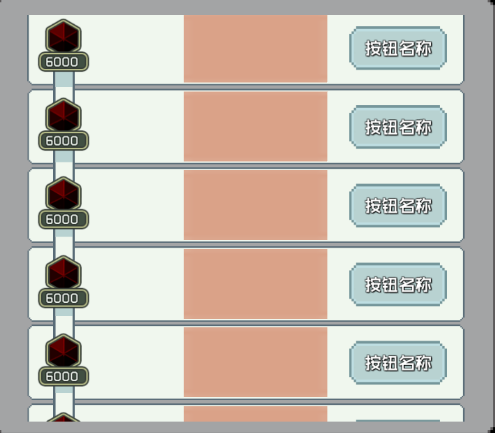``````--haveExp：当前拥有的
--ui.proto[index-1].exp：上一个item需要达到的
--ui.proto[index].exp：当前item需要达到的
--ui.proto[index+1].exp：下一个item需要达到的
--topSlider：上方进度
--botSlider：下方进度
-- 上面
local last = ui.proto[index-1] and ui.proto[index-1].exp or 0
if (haveExp - last) <= 0 then
topSlider.value = 0
elseif (haveExp - ui.proto[index].exp) >= 0 then
topSlider.value = 1
else
local total = (haveExp - last) / (ui.proto[index].exp - last)
topSlider.value = math.max(total-0.5,0)
end

--下面
if ui.proto[index+1] then
local _next = ui.proto[index+1].exp
if (ui.proto[index].exp - haveExp) >= 0  then
botSlider.value = 0
else
local total = (haveExp - ui.proto[index].exp) / ( _next - ui.proto[index].exp)
if total >= 0.5 then
botSlider.value = 1
else
botSlider.value = math.max(total,0)*2
end
end
end
``````

-----------------------------